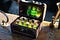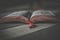# A Reference Notebook for (+30) Statistical Charts in Seaborn

A Reference Notebook for (+30) Statistical Charts in Seaborn | LinkedIn

The purpose of this tutorial is that we can build graphs to assist in the application of the data science process. We can employ visualizations during exploratory analysis, before or after processing data, construct statistical graphs to analyze datasets, identify variable relationships, or even to verify how data is distributed. We can do all this with Matplotlib, however, we have a library that is much better and much easier when we refer to statistical graphs — Seaborn.

Therefore, knowing how to create a visualization, regardless of which tool it…

# Como usar operações SQL no Pandas?

## Um guia de referência em SQL Join de DataFrames em Pandas

No último tutorial de operações úteis em Pandas, trabalhamos sempre com um único objeto, uma Series, DataFrame ou Array em NumPy — sempre um objeto. E se precisarmos trabalhar com mais de um objeto?

## Notebook de estudo

Acesse o Jupyter Notebook para consultar os conceitos que serão abordados sobre SQL Join em Pandas. Obs: as funções, outputs e termos importantes estão em negrito para facilitar a compreensão — pelo menos a minha.

## • Importar bibliotecas

Primeiro passo é importarmos o Pandas para podermos usar os pacotes, métodos e atributos, e o pacote NumPy para criarmos os Arrays:

`import pandas as pdimport numpy as np`

Para…

# A complete Exploratory Data Analysis with Python 🐍

A complete Exploratory Data Analysis guide with Python | LinkedIn

In this tutorial we’ll explore the rental dataset, perform transformations and reorganize the data as if we were actually preparing the data for modeling and creating models.

Visit Jupyter Notebook to see the concepts that will be covered about Exploratory Data Analysis. Note: important functions, outputs and terms are bold to facilitate understanding — at least mine.

It is common that we receive the data to solve any problem and need to analyze and explore data, seek relationships, seek how variables are organized, have or not the need to transform…

# Credit Card Operators Data Analysis with R

Anello | LinkedIn

In this article, we will address a very common case study, which aims to demonstrate some specific and relevant aspects of data analysis. In this analysis we will see how to work with categorical variables and the type of transformation that we must make in the variables to balance them, ending with the construction of a predictive model of Machine Learning throughout the study.

# The problem

We’ll work with a dataset that represents information from a credit card company. In the dataset are various information of customers who purchased card with the carrier and, the purpose of this analysis…

# Boost your Productivity and Joins using SQL with Pandas

Have you ever thought about using SQL with Pandas? | LinkedIn

In the last tutorial of useful operations in Pandas, we worked with a single object, a Series, DataFrame, or Array in NumPy — always an object. What if we need to work with more than one object?

## • Study notebook

Visit Jupyter Notebook to see the concepts that will be covered about SQL Join in Pandas. Note: Important functions, outputs, and terms are bold to facilitate understanding — at least mine.

## • Import libraries

First step is to import Pandas so we can use the packages, methods, and attributes, and the NumPy package to create…

# 🔟 Templates for Building Machine Learning Models in Python 🐍 (+Notebook)

## I bet you won’t regret it.Photo by Jan Ranft on Unsplash

We have seen the categories of supervised, unsupervised, and reinforcement learning — the main machine learning algorithms, and the process of building predictive models. Now we’ll see all of this in practice in the Python language through its main packages and build some machine learning models.

## Study Notebook

Visit Jupyter Notebook to see the concepts that will cover how to Build Machine Learning Models.

We will cover all preprocessing, machine learning algorithm training, model evaluation, and new data prediction during the composition of the Machine Learning template. The goal here is to address an introduction to the topic.

## Template for Building Machine Learning Models

This notebook contains a…

# 🔟 Templates for Building Machine Learning Models in Python 🐍 (with Notebook)

## I bet you won’t regret it.Photo by Wendy van Zyl from Pexels

We have seen before the categories of supervised, unsupervised, and reinforcement learning, the main machine learning algorithms, and the process of building predictive models. Now we’ll see all of this in practice in the Python language through its main packages and build some machine learning models.

## Study Notebook

Visit the Jupyter Notebook to see the concepts that will cover how to Building Machine Learning Models.

We will cover all preprocessing, machine learning algorithm training, model evaluation, and new data prediction during the composition of the Machine Learning template. The goal here is to address an introduction to the topic.

## Template for Building Machine Learning Models

This notebook contains…

# 🔟 Templates for Building Machine Learning Models in 🐍(with Notebook)

## I bet you won’t regret it.Photo by Wendy van Zyl from Pexels

We have seen before the categories of supervised, unsupervised, and reinforcement learning, the main machine learning algorithms, and the process of building predictive models. Now we’ll see all of this in practice in the Python language through its main packages and build some machine learning models.

## Study Notebook

Visit the Jupyter Notebook to see the concepts that will cover how to Building Machine Learning Models.

We will cover all preprocessing, machine learning algorithm training, model evaluation, and new data prediction during the composition of the Machine Learning template. The goal here is to address an introduction to the topic.

## Template for Building Machine Learning Models

This notebook contains…

# 10 Templates for Building Machine Learning Models with Notebook

## I bet you won’t regret it.Photo by Wendy van Zyl from Pexels

We have seen before the categories of supervised, unsupervised, and reinforcement learning, the main machine learning algorithms, and the process of building predictive models. Now we’ll see all of this in practice in the Python language through its main packages and build some machine learning models.

## Study Notebook

Visit the Jupyter Notebook to see the concepts that will cover how to Building Machine Learning Models.

We will cover all preprocessing, machine learning algorithm training, model evaluation, and new data prediction during the composition of the Machine Learning template. The goal here is to address an introduction to the topic.

## Template for Building Machine Learning Models

This notebook contains…

# 10 Templates for Building Machine Learning Models in Python (with Notebook)

## I bet you won’t regret it.Photo by Wendy van Zyl from Pexels

We have seen before the categories of supervised, unsupervised, and reinforcement learning, the main machine learning algorithms, and the process of building predictive models. Now we’ll see all of this in practice in the Python language through its main packages and build some machine learning models.

## Study Notebook

Visit the Jupyter Notebook to see the concepts that will cover how to Building Machine Learning Models.

We will cover all preprocessing, machine learning algorithm training, model evaluation, and new data prediction during the composition of the Machine Learning template. The goal here is to address an introduction to the topic.

## Template for Building Machine Learning Models

This notebook contains…## Anello

Composing a repository of books (i bought), authors (i follow) & blogs (direct ones) for my own understanding.